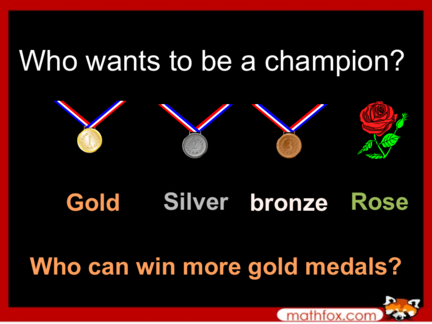Contributed by:1. Who wants to be a champion?
Gold Silver bronze Rose
Who can win more gold medals?
regrouping regrouping
3. 1 + 0 =
4. Bronze medal
3 + 0 =
5. Silver medal
8 + 0 =
6. Gold medal
9 + 0 =
7. 3 + 10 =
8. Bronze medal
4 + 10 =
9. Silver medal
7 + 10 =
10. Gold medal
9 + 10 =
11. 11 + 13 =
12. Bronze medal
12 + 10 =
13. Silver medal
14 + 13 =
14. Gold medal
17 + 12 =
15. 14 + 7 =
16. Bronze medal
14 + 8 =
17. Silver medal
15 + 5 =
18. Gold medal
13 + 19 =
19. Rose
2 + 3 + 1 =
20. Bronze medal
4 + 3 + 5 =
21. Silver medal
5 + 5 + 4 =
22. Gold medal
7 + 4 + 6 =